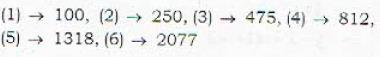Courses

# Differential Equations - 13

## 20 Questions MCQ Test Topic-wise Tests & Solved Examples for IIT JAM Mathematics | Differential Equations - 13

Description
This mock test of Differential Equations - 13 for Mathematics helps you for every Mathematics entrance exam. This contains 20 Multiple Choice Questions for Mathematics Differential Equations - 13 (mcq) to study with solutions a complete question bank. The solved questions answers in this Differential Equations - 13 quiz give you a good mix of easy questions and tough questions. Mathematics students definitely take this Differential Equations - 13 exercise for a better result in the exam. You can find other Differential Equations - 13 extra questions, long questions & short questions for Mathematics on EduRev as well by searching above.
QUESTION: 1

### The function f defined by f(x) - x [1 + 1/3 sin (log x2)], a ≠ 0,/(0) = 0 ([] represents the greatest integer function) is

Solution: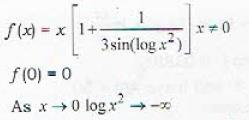and sin (-∞) can be any value between - 1 and 1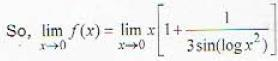The value of integral part can also become ∞, but in all cases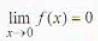due to factor x in it.
f'(x) does not exist because
f' (x) for x is 1 but integral value which is its coefficient changes to give different values.

QUESTION: 2

### If f is twice differentiable function such that f ''(x) = - f(x), f '(x) = g(x) and h(x) = [f(x)]2 + [g(x)]2, also if h(5) = 11, then h(10) is equal to

Solution: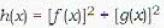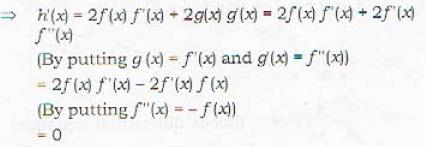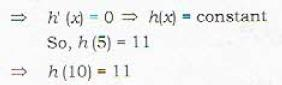QUESTION: 3

### The solution of the equation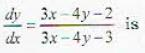Solution: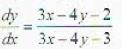........(i)
Let 3x - 4y = v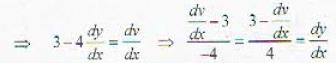Putting in given D.E. (i) we get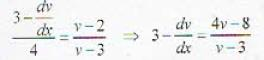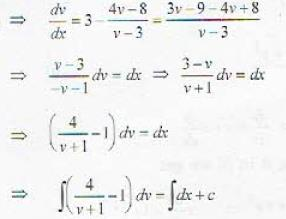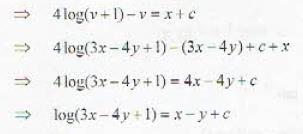QUESTION: 4

The solution of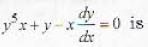Solution: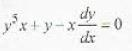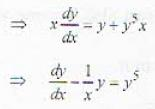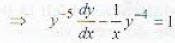.....(i)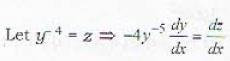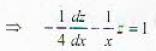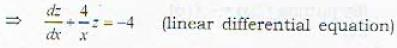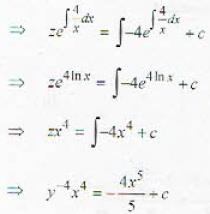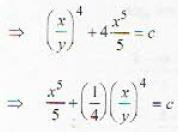QUESTION: 5

The solution of the differential equation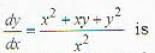Solution: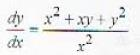....(i)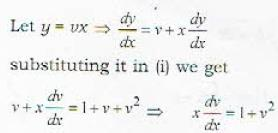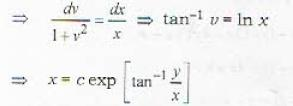QUESTION: 6

The solution of the differential equation y(2xy + ex)dx - exdy = 0 is

Solution:

y(2xy + ex)dx - exdy = 0  ....(0i)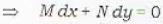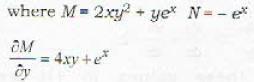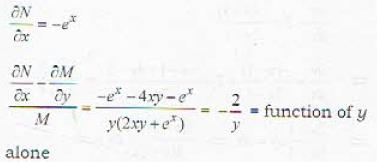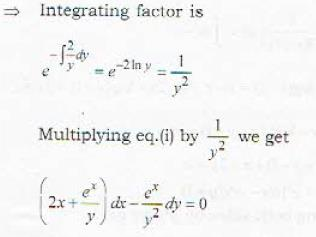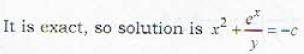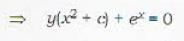QUESTION: 7

The solution of the differential equation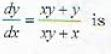Solution: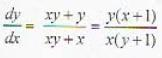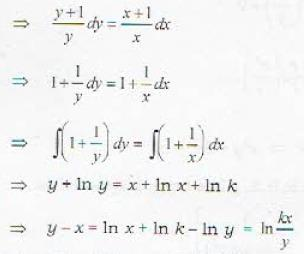QUESTION: 8

The half-life of a radioactive substance is the time required for one-half of that substance to decay. The amount of 11C, an isotope of carbon present at a future time t (in months) is given by A(t) = 100 exp [- 0.0338 t]. The half life of the material in months is

Solution: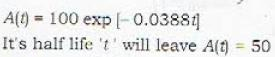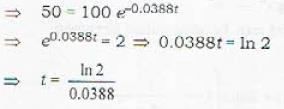QUESTION: 9

Consider a pair of differential equations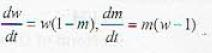Eliminating t in the two equations results in

Solution: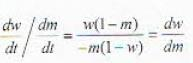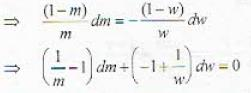QUESTION: 10

Consider L-C-R circuit which is governed by the differential equations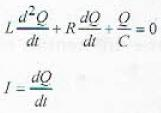Writing ω2 = (LC)-1, 2b =  RL-1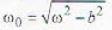and imposing initial condition Q(0) = Q0, l(0) = 0 leads to the current

Solution:

After substituting the given values we derive equation in option (4).

QUESTION: 11

The rare at which body changes temperature is proportional to the difference between its temperature and ihat of the surrounding medium. This is called Newton's law of cooling. If y = f{t} is the unknown temperature of The body at time t and M(t) denotes the known temperature of the surrounding medium, Newton's law' leads to the differential equation where k is a positive constant.

Solution: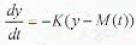QUESTION: 12

tan-1 x + tan-1 y = c is the general solution of the differential equation

Solution: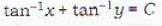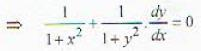(By differentiating)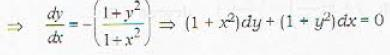QUESTION: 13

The differential equation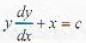represents

Solution: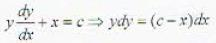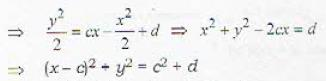QUESTION: 14

If u = log (x3 + y2 + z3 - 3xyz), then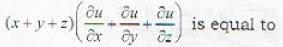Solution:

u = log(x3 + y3 + z3 - 3xyx)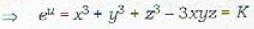As K is a homogeneous function of degree 3, So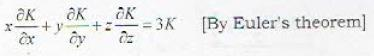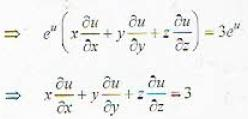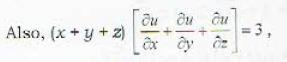As rest terms will cancel by symmetricity.

QUESTION: 15

The solution of the differential equal ion (1 + x2) dy/dx = (1 + y2) is

Solution: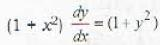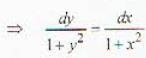......(i)
Integrating both sides of eq. (i)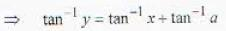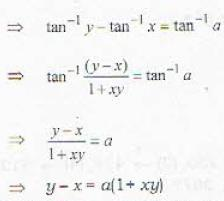QUESTION: 16

If a population grows at the rate of 5% per year, it will double (in years) after

Solution:

After n years population will be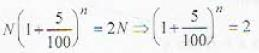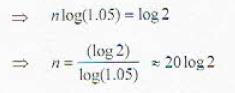QUESTION: 17

The differential equation of all circles passing through the origin and having their centres on the y-axis is

Solution: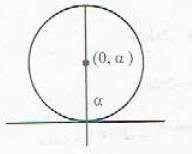x2 + (y - a)2 = a2    .....(1)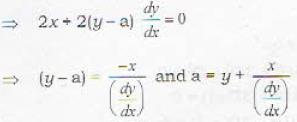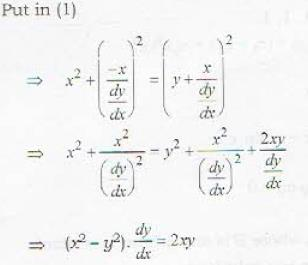QUESTION: 18

The geometrical interpretation of the graph of the differential equation: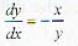is a family of

Solution: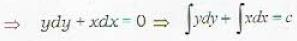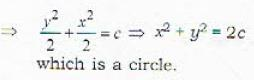QUESTION: 19

The solution curves of the given differential equation: xdx - dy = 0 constitute a family of

Solution: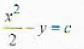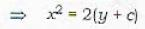QUESTION: 20

If left to grow undisturbed, the fish population in a lake would be 50 per cent more than it was in the previous year. However, for research purposes 100 fishes are added to the population each year. If the initial population of fish in the pond was zero then how many years the slocking program should continue till the population increases to 2000?

Solution: